# RD Sharma Solutions Class 9 Maths Chapter 17 Constructions Exercise 17.3

Exercise 17.3 of RD Sharma textbook solutions are given here. Solutions to the exercise 17.3 in RD Sharma class 9 textbook for the chapter of Constructions is given in the below link. Check these detailed solutions to understand the in-depth concepts of Constructions of different types of triangles. Students can find a lot more exercises on various subjects in RD Sharma Class 9 Solutions, which are given in a detailed manner for their ease.

## Download PDF of RD Sharma Solutions for Class 9 Maths Chapter 17 Construction Exercise 17.3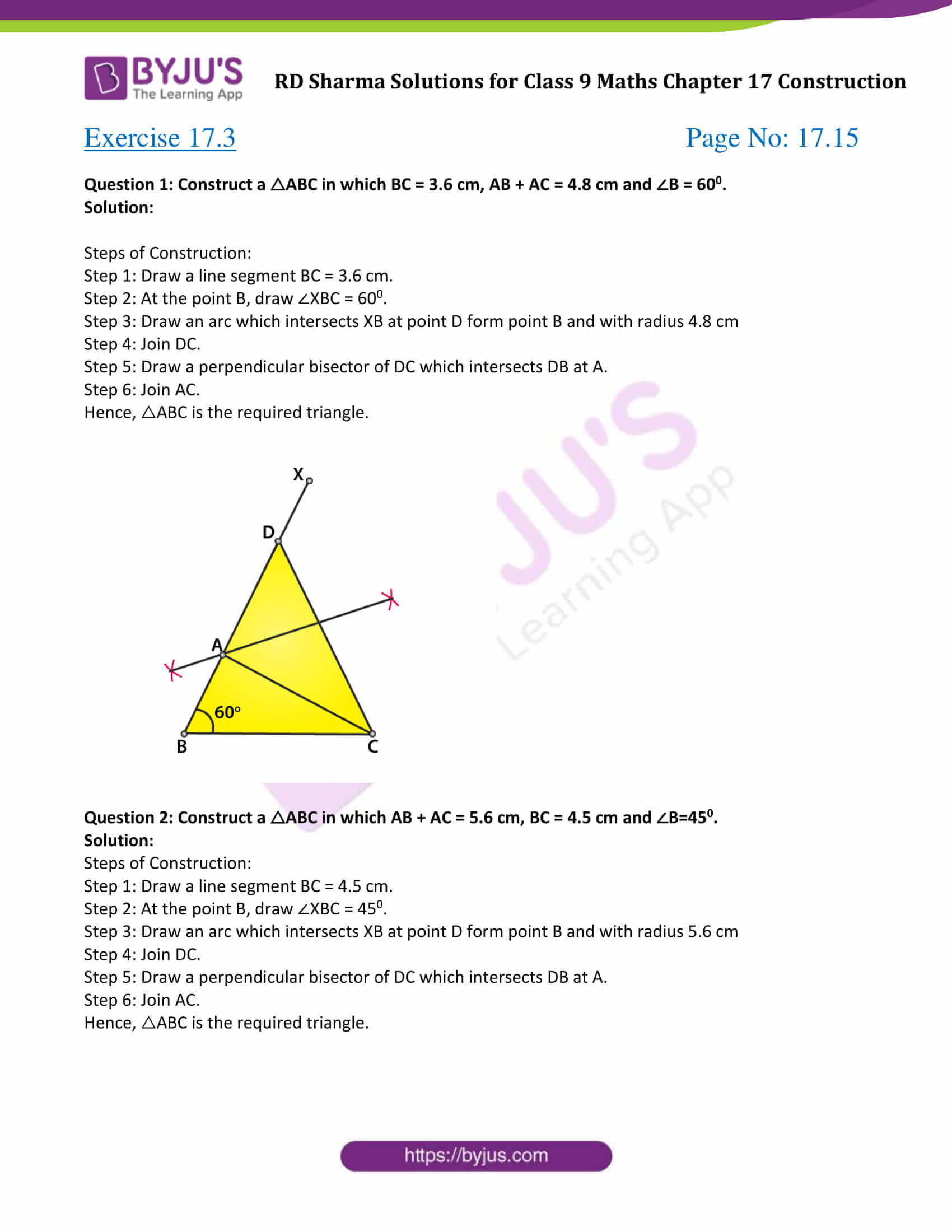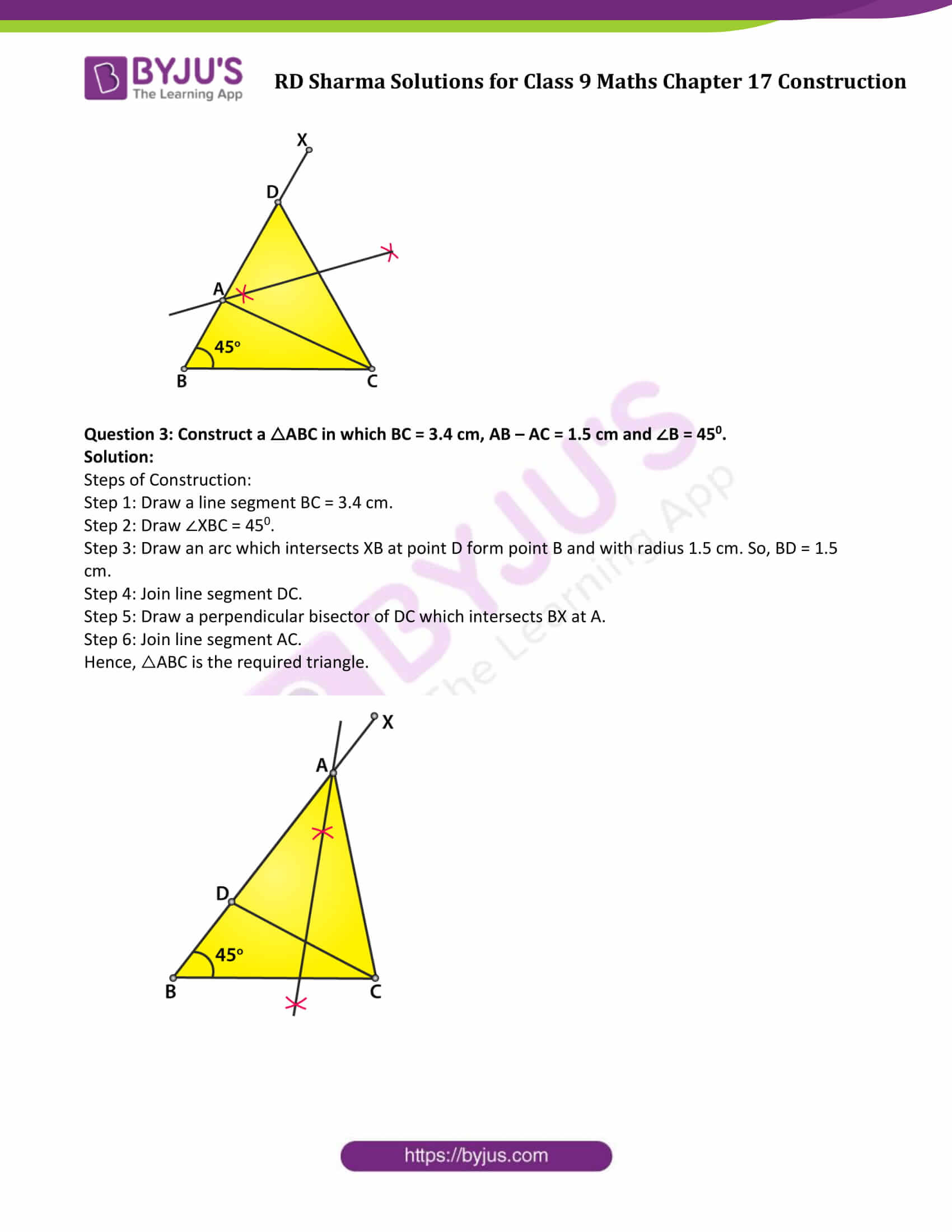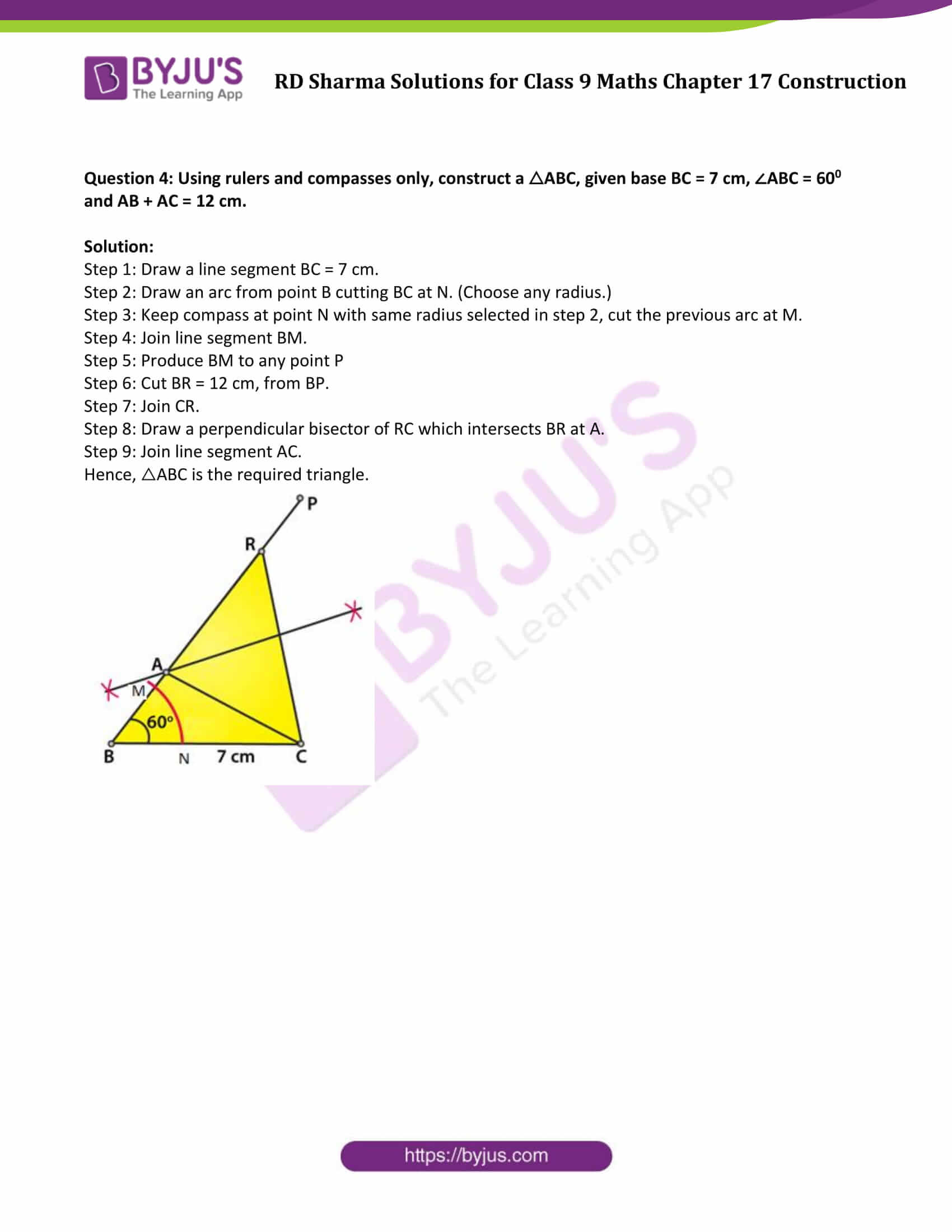### Access Answers to Maths RD Sharma Solutions for Class 9 Chapter 17 Construction Exercise 17.3 Page number 17.15

Question 1: Construct a △ABC in which BC = 3.6 cm, AB + AC = 4.8 cm and ∠B = 600.

Solution:

Steps of Construction:

Step 1: Draw a line segment BC = 3.6 cm.

Step 2: At the point B, draw ∠XBC = 600.

Step 3: Draw an arc which intersects XB at point D form point B and with radius 4.8 cm

Step 4: Join DC.

Step 5: Draw a perpendicular bisector of DC which intersects DB at A.

Step 6: Join AC.

Hence, △ABC is the required triangle.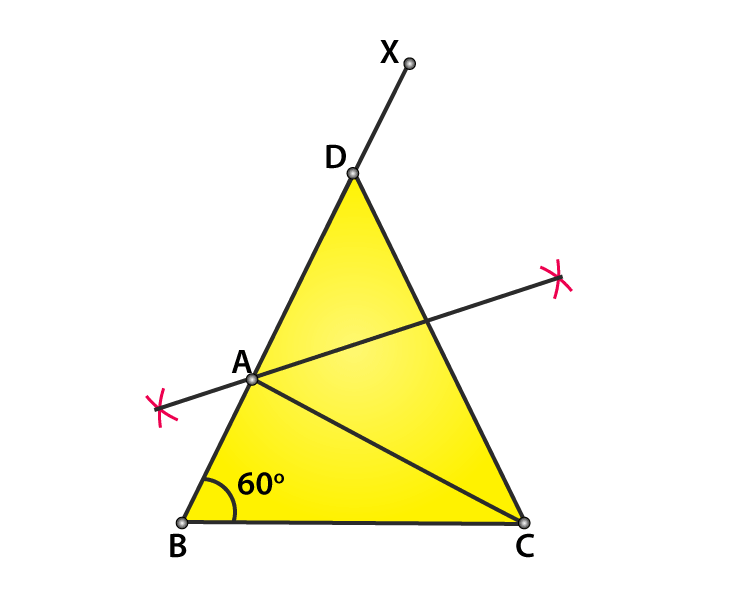Question 2: Construct a △ABC in which AB + AC = 5.6 cm, BC = 4.5 cm and ∠B=450.

Solution:

Steps of Construction:

Step 1: Draw a line segment BC = 4.5 cm.

Step 2: At the point B, draw ∠XBC = 450.

Step 3: Draw an arc which intersects XB at point D form point B and with radius 5.6 cm

Step 4: Join DC.

Step 5: Draw a perpendicular bisector of DC which intersects DB at A.

Step 6: Join AC.

Hence, △ABC is the required triangle.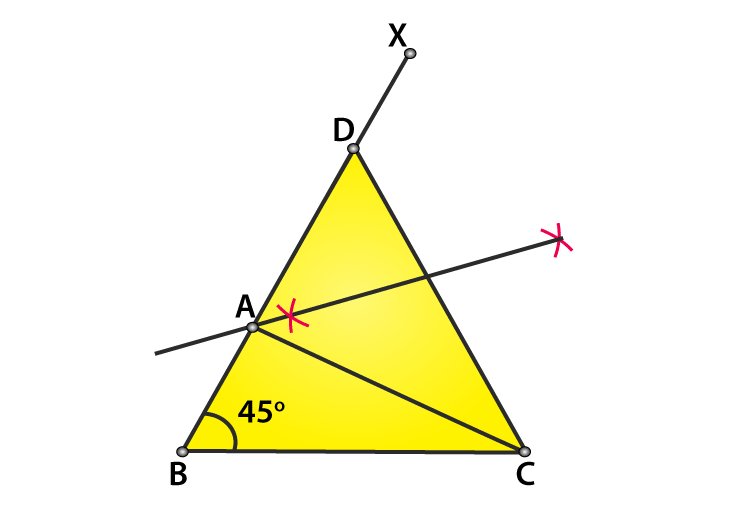Question 3: Construct a △ABC in which BC = 3.4 cm, AB – AC = 1.5 cm and ∠B = 450.

Solution:

Steps of Construction:

Step 1: Draw a line segment BC = 3.4 cm.

Step 2: Draw ∠XBC = 450.

Step 3: Draw an arc which intersects XB at point D form point B and with radius 1.5 cm. So, BD = 1.5 cm.

Step 4: Join line segment DC.

Step 5: Draw a perpendicular bisector of DC which intersects BX at A.

Step 6: Join line segment AC.

Hence, △ABC is the required triangle.Question 4: Using rulers and compasses only, construct a △ABC, given base BC = 7 cm, ∠ABC = 600 and AB + AC = 12 cm.

Solution:

Step 1: Draw a line segment BC = 7 cm.

Step 2: Draw an arc from point B cutting BC at N. (Choose any radius.)

Step 3: Keep compass at point N with same radius selected in step 2, cut the previous arc at M.

Step 4: Join line segment BM.

Step 5: Produce BM to any point P

Step 6: Cut BR = 12 cm, from BP.

Step 7: Join CR.

Step 8: Draw a perpendicular bisector of RC which intersects BR at A.

Step 9: Join line segment AC.

Hence, △ABC is the required triangle.## RD Sharma Solutions for Class 9 Maths Chapter 17 Construction Exercise 17.3

RD Sharma Solutions Class 9 Maths Chapter 17 Construction Exercise 17.3 is based on the following topics:

• Constructions of Triangles
• Construction of an Equilateral Triangle
• Construction of a triangle when its base, sum of the other two sides and one base angle are given
• Construction of a triangle when its base, difference of the other two sides and one base angle are given
• Construction of a triangle of given perimeter and two base angles